# NCERT Solutions for Class 7 Maths Chapter 2: Fractions and Decimals

In this page we have NCERT Solutions for Class 7 Maths Chapter 2: Fractions and Decimals . Hope you like them and do not forget to like , social share and comment at the end of the page.

## Exercise 2.1

Question 1
Solve
i. 2 – 3/5
ii. 4 + 7/8
iii. 3/5 + 2/7
iv. 9/11 – 4/15
v. 7/10 + 2/5 + 3/2
vi. $2 \frac {2}{3}+ 3 \frac{1}{2}$
vii. $8 \frac {1}{2} – 3 \frac{5}{8}$
Solution
We  have to convert them to Like Fraction to perform these addition and substraction
i. 2 – 3/5
= (2 × 5)/5 – 3/5
= 10/5 – 3/5
= (10 – 3) ÷ 5 = 7/5
ii. 4 + 7/8
= (4 × 8)/8 + 7/8
= 32/ 8 + 7/8
= (32 + 7)/8 = 39/8
iii. 3/5 + 2/7
= (3 × 7) ÷ (5 × 7) + (2 × 5) ÷ (7 × 5)
= 21/35 + 10/35
= (21 + 10) ÷ 35 = 31/35
iv. 9/11 – 4/15
= (9 × 15) ÷ (11 × 15) – (4 × 11) ÷ (15 × 11)
= 135/165 – 44/165
= (135 – 44) ÷ 165 = 91/ 165
v. 7/10 + 2/5 + 3/2
= 7/10 + (2 × 2) ÷ (5 × 2) + (3 × 5) ÷ (2 × 5)
= 7/ 10 + 4/ 10 + 15/10
= (7 + 4 + 15) ÷10
= 26/ 10 = 13/5 = 2 3/5
vi. $2 \frac {2}{3}+ 3 \frac{1}{2}$
= 8/3 + 7/2
= (8 × 2) ÷ (3 × 2) + (7 × 3) ÷ (2 × 3)
= 16/6 + 21/ 6
= (16 + 21) ÷ 6 = 37/6 = $6 \frac{1}{6}$
vii. $8 \frac {1}{2} – 3 \frac{5}{8}$
= 17/2 – 29/8
= (17 × 4) ÷ (2 × 4) – 29/ 8
= 68/8 – 29/8
= (68 – 29) ÷ 8 = 39/8 = $4 \frac{7}{8}$
Question 2
Arrange the following in descending order:
i. 2/9, 2/3, 8/21
ii. 1/5, 3/7, 6/10
Solution
i. 2/9, 2/3, 8/21
Changing the fractions into like fractions, we get
(2 × 4) ÷ (9 × 4), (2 × 4) ÷ (3 × 4), 8/ 21
8/ 36, 8/12, 8/21
8/12 > 8/21 > 8/36
Therefore, 2/3 > 8/21 > 2/9
ii. 1/5, 3/7, 6/10
Changing the fractions into like fractions we get
(1 × 7 × 10) ÷ (5 × 7 × 10), (3 × 5 × 10) ÷ (7 × 5 × 10), (7 × 7 × 10) ÷ (10 × 7 × 5)
70/350, 150/350, 245/350
245/350 > 150/350 > 70/350
Therefore, 7/10 > 3/7 > 1/5
Question 3
In a “magic square”, the sum of the numbers in each row, in each column and along the diagonals is the same. Is this a magic square?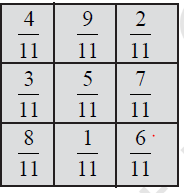Solution:
Sum, along first row = 4/11 + 9/11 + 2/11
= (4 + 9 + 2) ÷ 11 = 15/11
Sum, along second row = 3/11 + 5/11 + 7/11
= (3 + 5 + 7) ÷ 11 = 15/11
Sum, along third row = 8/11 + 1/11 + 6/11
= (8 + 1 + 6) ÷ 11 = 15/11
Sum, along first column = 4/11 + 3/11 + 8/11
= (4 + 3 + 8) ÷ 11 = 15/11
Sum, along second column = 9/11 + 5/11 + 1/11
= (9 + 5 + 1) ÷ 11 = 15/11
Sum, along third column = 2/11 + 7/11 + 6/11
= (2 + 7 + 6) ÷ 11 = 15/11
Sum, along first diagonal = 6/11 + 5/11 + 4/11
= (6 + 5 + 4) ÷ 11 = 15/11
Sum, along second diagonal = 8/11 + 5/11 + 2/11
= (8 + 5 + 2) ÷ 11 = 15/11
In this square, the sum of each row, column and diagonal are same. So, this is a magic square
Question 4
A rectangular sheet paper is  $12 \frac {1}{2}$  long  and $10\frac {2}{3}$ cm wide. Find its perimeter.
Solution:
Length of the rectangular sheet of paper = $12 \frac {1}{2}$        cm
Breadth of the rectangular sheet of paper = $10\frac {2}{3}$  cm
Perimeter of the rectangular sheet of paper = 2 × (length + breadth)
$=2 X[ 12 \frac {1}{2} + 10\frac {2}{3}]$
= 2 × (25/2 + 32/3)
Converting into equivalent Fractions
= 2 × (75/6 + 64/6)
= 2 × [ (75 + 64) ÷ 6]
= 2 × (139/6)
= (2 × 139) ÷ 6 = 139/3 = $46 \frac {1}{3}$ cm
Question 5
Find the perimeter of
i) ? ABE
ii)  the rectangle BCDE in this figure. Whose perimeter is greater?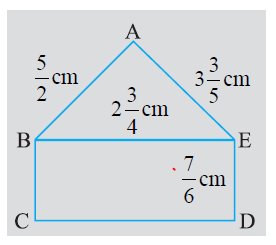Solution
i. The perimeter of ? ABE = AB + BE + EA
$= \frac {5}{2} + 2 \frac{3}{4} + 3 \frac{3}{4}$
= 5/2 + 11/4 + 18/5
Converting into equivalent fractions
= 100/40 + 144/40 + 110/40
= (100 + 144 + 110) ÷ 40
= 354/40 = 177/20 cm
ii. The length of rectangle =   $2 \frac{3}{4}$    cm
The breadth of rectangle = 7/6 cm
Perimeter of the rectangle = 2 × (length + breadth)
$=2 X (2 \frac {3}{4} + \frac {7}{6})$
= 2 × (11/4 + 7/6)
= 2 × (66/24 + 28/24)
= 2 × [ (66 + 28) ÷ 24]
= 2 × 94/24 = 47/6 cm
Therefore, the perimeter of the rectangle BCDE is 47/6 cm
Perimeter of the triangle ? ABE = 177/20 cm
Perimeter of the rectangle BCDE = 47/6 cm
Changing the fractions into like fractions, we get
177/20, 47/6
(177 × 6) ÷ (20 × 6), (47 × 20) ÷ (6 × 20)
1062/120, 940/120
1062/ 120 > 940/120
Therefore, 177/20 or 8 17/20 is greater
So, the perimeter of ? ABE is greater than the perimeter of rectangle BCDE.
Question 6
Salil wants to put a picture in a frame. The picture is $7 \frac {3}{5}$ cm wide. To fit in the frame the picture cannot be more than   $7\frac {3}{10}$ cm wide. How much should the picture be trimmed?
Solution
Width of picture =$7 \frac {3}{5}$ cm
Required width of picture =  $7\frac {3}{10}$ cm
The picture should be trimmed by
$=7 \frac {3}{5} - 7 \frac{3}{10}$
= 38/5 – 73/10
= 76/10 – 73/10 = (76 – 73) ÷ 10 = 3/10 cm
Question 7
Ritu ate 3/5 part of an apple and the remaining apple was eaten by her brother Somu. How much part of the apple did Somu eat? Who had the larger share? By how much?
Solution
The part of apple eaten by Ritu = 3/5
The part of apple eaten by Somu = 1 – part of apple eaten by Ritu
= 1 – 3/5
= 2/5
Therefore, Somu ate 2/5 part of the apple.
Since, 3/5  > 2/5 , Ritu had the larger share.
Difference between the two shares 3/2 – 2/5 = (3 – 2) ÷ 5 = 1/5
Question 8
Michael finished coloring a picture in 7/12 hour. Vaibhav finished coloring the same picture in 3/4 hour. Who worked longer? By what fraction was it longer?
Solution
Time taken by Michael = 7/12 hour
Time taken by Vaibhav = 3/4 hour
Changing the fractions into like fractions, we get
7/12, 3/4
7/12, (3 × 3) ÷ (4 × 3)
7/12, 9/12
Since, 9/12 > 7/12, Vaibhav worked longer.
Difference = 9/12 – 7/12 = (9 – 7) ÷ 12 = 2/12 = 1/6 hours longer

## Exercise 2.2

Question 1
1. Which of the drawings a) to d)
show:
i. 2 × (1/5)
ii. 2 × (1/2)
iii. 3 × (2/3)
iv. 3 × (1/5)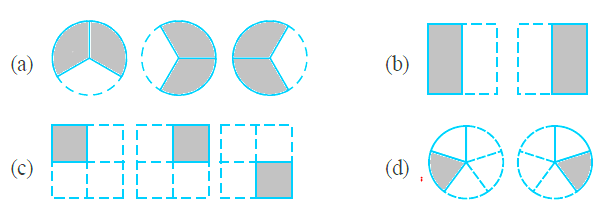Solutions
i) d
ii) b
iii) a
iv) c
Question 2
Some pictures a) to c) are given below. Tell which of them show:
i. 3 × (1/5) = 3/5
ii. 2 × (1/3) = 2/3
iii. 3 × (3/4) = 2 1/4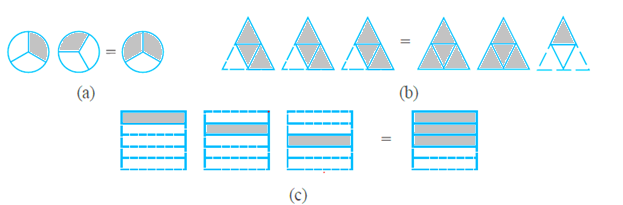Solutions
i)c
ii) a
iii) b
Question 3
Multiply and reduce lowest form and convert into a Mixed Fraction.
i. 7 × (3/5)
ii. 4 × (1/3)
iii. 2 × (6/7)
iv. 5 × (2/9)
v. (2/3) × 4
vi. (5/2) × 6
vii. 11 × (4/7)
viii. 20 × (4/5)
ix. 13 × (1/3)
x. 15 × (3/5)
Solutions
i. 7 × (3/5) = (3 × 7) ÷ 5 = 21/5 =  $4 \frac {1}{5}$
ii. 4 × (1/3) = (4 × 1) ÷ 3 = 4/3 = $1 \frac{1}{3}$
iii. 2 × (6/7) = (2 × 6) ÷ 7 = 12/7 =$1 \frac {5}{7}$
iv. 5 × (2/9) = (5 × 2) ÷ 9 = 10/9 =$1 \frac {1}{9}$
v. (2/3) × 4 = (2 × 4) ÷ 3 = 8/3 =  $2 \frac{2}{3}$
vi. (5/2) × 6 = (5 × 6) ÷ 2 = 30/2 = 15
vii. 11 × (4/7) = (11 × 4) ÷ 7 = 44/7 =$6 \frac {2}{7}$
viii. 20 × (4/5) = (20 × 4) ÷ 5 = 80/5 = 16
ix. 13 × (1/3) = (13 × 1) ÷ 3 = 13/3 =  $4 \frac{1}{3}$
x. 15 × (3/5) = (15 × 3) ÷ 5 = 45/5 = 9
Question 4
i. 1/2 of the circles in box a)
ii. 2/3 of the triangles in box b)
iii. 3/5 of the squares in box c)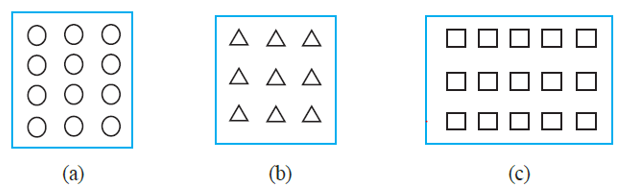Solutions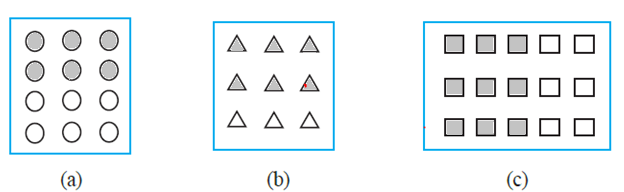Question 5
Find
a. 1/2 of i. 24, ii. 46
b. 2/3 of i. 18, ii. 27
c. 3/4 of i. 16, ii. 36
d. 4/5 of i. 20, ii. 35
Solutions
a. 1/2 of i. 24, ii. 46
i. 1/2 of 24 = (1/2) × 24 = 12
ii. 1/2 of 46 = (1/2) × 46 = 23
b. 2/3 of i. 18, ii. 27
i. 2/3 of 18 = (2/3) × 18 = 12
ii. 2/3 of 27 = (2/3) × 27 = 18
c. 3/4 of i. 16, ii. 36
i. 3/4 of 16 = (3/4) × 16 = 12
ii. 3/4 of 36 = (3/4) × 36 = 27
d. 4/5 of i. 20, ii. 35
i. 4/5 of 20 = (4/5) × 20 = 16
ii. 4/5 of 35 = (4/5) × 35 = 28
Question 6
Multiply and express as a mixed fraction
a. 3 ×  $5 \frac {1}{5}$
b. 5 × $6 \frac{3}{4}$
C. 7 × $2 \frac {1}{4}$
d. 4 ×  $6 \frac{1}{3}$
e. $3 \frac {1}{4}$ × 6
f.$3 \frac {2}{5}$ × 8
Solutions
a.3 ×  $5 \frac {1}{5}$= 3 × (26/5) = 78/5 = $15 \frac{3}{5}$
b. 5 × $6 \frac{3}{4}$= 5 × 27/4 = 135/4 =  $33 \frac{3}{4}$
C. 7 × $2 \frac {1}{4}$= 7 × 9/4 = 63/4 =  $15 \frac {3}{4}$
d. 4 ×  $6 \frac{1}{3}$= 4 × 19/3 = 76/3 = $25 \frac {1}{2}$
e. $3 \frac {1}{4}$ × 6 = 13/4 × 6 = 78/4 =  $19 \frac{2}{4}$
f. $3 \frac {2}{5}$ × 8= 17/5 × 8 = 136/5 = $27 \frac {1}{5}$
Question 7
7. Find
a. 1/2 of i.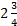, ii.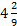b. 5/8 of i.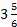, ii.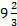Solutions
a. 1/2 of i., ii.i. 1/2 of= 1/2 × 11/4 = 11/8 =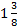ii. 1/2 of= 1/2 × 38/9 = 38/18 = 19/9 =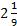b. 5/8 of i., ii.i. 5/8 of= 5/8 × 23/6 = 115/48 =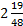ii. 5/8 of= 5/8 × 29/3 = 145/24 =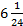Question 8
Vidya and Pratap went for a picnic. Their mother gave them a water bottle that contained 5 litres of water. Vidya consumed 2/3 of the water. Pratap consumed the remaining water.
i. How much water did Vidya drink?
ii. What Fraction of the total quantity of water did Pratap drink?
Solution
i. Water consumed by Vidya = 2/5 of 5 litres = 2/5 × 5 = 2 litres
ii. Water consumed by Pratap = 1 – 2/5 = (5 – 2) ÷ 5 = 3/5 of the total water
Therefore, the fraction of total quantity of water = 3/5

## Exercise 2.3

Question 1
1. i. 1/4 of a. 1/4, b. 3/5, c. 4/3
ii. 1/7 of a. 2/9, b. 6/5, c. 3/10
Solution
1. i. a.1/4 of 1/4
1/4 × 1/4 = (1 × 1) ÷ (4 × 4) = 1/16
b. 1/4 of 3/5
1/4 × 3/5 = (1 × 3) ÷ (4 × 5) = 3/20
c. 1/4 of 4/3
1/4 × 4/3 = (1 × 4) ÷ (4 × 3) = 1/3
ii. a. 1/7 0f 2/9
1/7 × 2/9 = (1 × 2) ÷ (7 × 9) = 2/63
b. 1/7 of 6/5 = (1 × 6) ÷ (7 × 5) = 6/35
c. 1/7 of 3/10
1/7 × 3/10 = (1 × 3) ÷ (7 × 10) = 3/70
Question 2
Multiply and reduce to lowest form (if possible);
i. 2/3 × $2 \frac {2}{3}$
ii. 2/7 × 7/9
iii. 3/8 × 6/4
iv. 9/5 × 3/5
v. 1/3 × 15/8
vi. 11/2 × 3/10,
vii. 4/5 × 12/7
Solutions
i. 2/3 × $2 \frac {2}{3}$
=2/3 × 8/3 = (2 × 8) ÷ (3 × 3) = 16/9 =  $1 \frac {7}{9}$
ii. 2/7 × 7/9
2/7 × 7/9 = (2 × 7) ÷ (7 × 9) = 2/9
iii. 3/8 × 6/4
3/8 × 6/4 = (3 × 6) ÷ (8 × 4) = (3 × 3) ÷ (4 × 4) = 9/16
iv. 9/5 × 3/5
9/5 × 3/5 = (9 × 3) ÷ (5 × 5) = 27/25 = $1 \frac {2}{25}$
v. 1/3 × 15/8
1/3 × 15/8 = (1 × 15) ÷ (3 × 8) = (1 × 5) ÷ (1 × 8) = 5/8
vi. 11/2 × 3/10
11/2 × 3/10 = (11 × 3) ÷ (2 × 10) = 33/20 = $1 \frac {13}{20}$
vii. 4/5 × 12/7
4/5 × 12/7 = (4 × 12) ÷ (5 × 7) = 48/35 =  $1 \frac {13}{35}$
Question 3
Multiply the following fractions
i. 2/5 × $5 \frac {1}{4}$
ii.  $6 \frac {2}{5}$ × 7/9
iii. 3/2 × $5 \frac {1}{3}$
iv. 5/6 ×  $2 \frac {3}{7}$
v. $3 \frac {2}{5}$ × 4/7
vi. $2 \frac {3}{5}$ × 3
vii. $3 \frac {4}{7}$ × 3/5
Solutions
i. 2/5 × $5 \frac {1}{4}$
=2/5 × 21/4 = (2 × 21) ÷ (5 × 4) = (1 × 21) ÷ (5 × 2) = 21/10 =$2 \frac {1}{10}$
ii. $6 \frac {2}{5}$ × 7/9
=32/5 × 7/9 = (32 × 7) ÷ (5 × 9) = 224/45 = $4 \frac {44}{45}$
iii. 3/2 × $5 \frac {1}{3}$
= (3 × 16) ÷ (2 × 3) = (1 × 8) ÷ (1 × 1) = 8
iv.  5/6 ×  $2 \frac {3}{7}$
5/6 × 17/7 = (5 × 17) ÷ (6 × 7) = 85/42 =  $2 \frac {1}{42}$
v. $3 \frac {2}{5}$ × 4/7= 17/5 × 4/7 = (17 × 4) ÷ (5 × 7) = 68/35 = $1 \frac {33}{35}$
vi. $2 \frac {3}{5}$ × 3
=13/5 × 3 = (13 × 3) ÷ 5 = 39/5 =  $7 \frac {4}{5}$
vii. $3 \frac {4}{7}$   × 3/5
=25/7 × 3/5 = (25 × 3) ÷ (7 × 8) = (5 × 3) ÷ (7 × 1) = 15/7 = $2 \frac {1}{7}$

Question 4
4. Which is greater:
i. 2/7 of 3/4 or 3/5 of 5/8
ii. 1/2 of 6/7 or 2/3 of 3/7
Solutions
i. 2/7 of 3/4 or 3/5 of 5/8
2/7 of 3/4
2/7 × 3/4 = (2 × 3) ÷ (7 × 4) = (1 × 3) ÷ (7 × 2) = 3/14
3/5 of 5/8
3/5 × 5/8 = (3 × 5) ÷ (5 × 8) = (3 × 1) ÷ (1 × 8) = 3/8
3/14, 3/8
3/8 > 3/14
Therefore, 3/5 of 5/8 > 2/7 of 3/4
ii. 1/2 of 6/7 or 2/3 of 3/7
1/3 of 6/7
1/2 × 6/7 = (1 × 6) ÷ (2 × 7) = 3/7
2/3 of 3/7
2/3 × 3/7 = (2 × 3) ÷ (3 × 7) = 2/7
3/7. 2/7
3/7 > 2/7
Therefore, 1/2 of 6/7 > 2/3 of 3/7
Question 5
Saili plants 4 saplings, in a row, in her garden. The distance between two adjacent saplings is 3/4 m. Find the distance between the first and the last sapling.
Solution:
Gaps between first and last saplings = 3 times Length of one gap
Therefore, distance between first and last sapling = 3 × 3/4 = 9/4 =  $2 \frac {1}{4}$ m
Question 6
Lipika reads a book for $1 \frac {3}{4}$ hours every day. She reads the entire book in 6 days. How many hours in all were required by her to read the book?
Solution:
Number of hours Lupica reads the book per day =   $1 \frac {3}{4}$
Number of days = 6
Total number of hours required by Lipika to read the book = $1 \frac {3}{4}$ × 6 = 7/4 × 6 = 42/4 = 21/2 =  $10 \frac {1}{2}$ hours
Question 7
A car runs 16 km using 1 litre of petrol. How much distance will it cover usinglitres of petrol?
Solution:
Car can run one-liter petrol = 16 Km
Number of Km a car can run forlitres petrol =X 16 = 11/4 × 16 = 11 × 4 = 44 km
Question 8
a.
i. Provide the number in the box, such that 2/3 × _____ = 10/30.
ii. The simplest form of the number obtained in box above  is ______
b.
i. Provide the number in the box , such that 3/5 × ______ = 24/75
ii. The simplest form of the number obtained in box  is ______
Solution
a. i. 2/3 × 5/10 = 10/30.
ii. The simplest form of the number obtained in 5/10 is 1/2
b. i. 3/5 × 8/15 = 24/75
ii. The simplest form of the number obtained in 8/15 is 8/15.

## Exercise 2.4

Question 1
1. Find:
i. 12 ÷ (3/4)
ii. 14 ÷ (5/6)
iii. 8 ÷ (7/3)
iv. 4 ÷ 8/3
v. 3 ÷ $2 \frac {1}{3}$
vi. 5 ÷ $3 \frac {4}{7}$
Solution
i. 12 ÷ (3/4)
12 × 4/3 = (12 × 4) ÷ 3 = 4 × 4 = 16
ii. 14 ÷ (5/6)
14 × 6/5 = (14 × 6) ÷ 5 = 84 ÷ 5 = 84/5 =$16 \frac {4}{5}$
iii. 8 ÷ (7/3)
8 × 3/7 = (8 × 3) ÷ 7 = 24 ÷ 7 = 24/7 = $3 \frac {3}{7}$
iv. 4 ÷ 8/3
4 × 3/8 = (4 × 3) ÷ 8 = 3 ÷ 2 = 3/2 =$1 \frac {1}{2}$
v.  3 ÷ $2 \frac {1}{3}$= 3 ÷ 7/3
3 × 3/7 = (3 × 3) ÷ 7 = 9 ÷ 7 = 9/7 =$1 \frac {2}{7}$
vi. 5 ÷ $3 \frac {4}{7}$= 5 ÷ 25/7
5 × 7/25 = (5 × 7) ÷ 25 = (1 × 7) ÷ 5 = 7/5 =$1 \frac {2}{5}$
Question 2
2. Find the reciprocal of each of the following fractions. Classify the reciprocals as Proper Fractions, Improper Fractions and Whole Numbers.
i. 3 ÷ 7
ii. 5 ÷ 8
iii. 9 ÷ 7
iv. 6 ÷ 5
v. 12 ÷ 7
vi. 1 ÷ 8
vii. 1 ÷ 11
Solution
i. 3 ÷ 7 = 3/7
Reciprocal of 3/7 is 7/3. 7/3 is an improper fraction.
ii. 5 ÷ 8 = 5/8
Reciprocal of 5/8 is 8/5. 8/5 is an improper fraction.
iii. 9 ÷ 7 = 9/7
Reciprocal of 9/7 is 7/9. 7/9 is a proper fraction.
iv. 6 ÷ 5 = 6/5
Reciprocal of 6/5 is 5/6. 5/6 is a proper fraction.
v. 12 ÷ 7 = 12/7
Reciprocal of 12/7 is 7/12. 7/12 is a proper fraction.
vi. 1 ÷ 8 = 1/8
Reciprocal of 1/8 is 8. 8 is a whole number.
vii. 1 ÷ 11 = 1/11
Reciprocal of 1/11 is 11. 11 is a whole number.
Question 3
Find
i. 7/3 ÷ 2
ii. 4/9 ÷ 5
iii. 6/13 ÷ 7
iv. $4 \frac {1}{3}$ ÷ 3
v. $3 \frac {1}{2}$ ÷ 4
vi. $4 \frac{3}{7}$ ÷ 7
Solutions
i. 7/3 ÷ 2
7/3 ÷ 2/1 = 7/3 × 1/2 = (7 × 1) ÷ (3 × 2) = 7/6
ii. 4/9 ÷ 5
4/9 ÷ 5/1 = 4/9 × 1/5 = (4 × 1) ÷ (9 × 5) = 4/45
iii. 6/13 ÷ 7
6/13 ÷ 7/1 = 6/13 × 1/7 = (6 × 1) ÷ (13 × 7) = 6/91
iv. $4 \frac {1}{3}$ ÷ 3 = 13/3 ÷ 3
13/3 ÷ 3/1 = 13/3 × 1/3 = (13 × 1) ÷ (3 × 3) = 13/9
v. $3 \frac {1}{2}$ ÷ 4 = 7/2 ÷ 4
7/2 ÷ 4/1 = 7/3 × 1/4 = (7 × 1) ÷ (2 × 4) = 7/8
vi. $4 \frac{3}{7}$ ÷ 7 = 31/7 ÷ 7
31/7 ÷ 7/1 = 31/7 × 1/7 = (31 × 1) ÷ (7 × 7) = 31/49
Question 4
Find
i. 2/5 ÷ ½
ii. 4/9 ÷ 2/3
iii. 3/7 ÷ 8/7
iv. $2 \frac {1}{3}$  ÷ 3/5
v. $3 \frac {1}{2}$ ÷ 8/3
vi. 2/5 ÷  $1 \frac {1}{2}$
vii. $3 \frac {1}{5}$ ÷ $1 \frac {2}{3}$
viii. $2 \frac {1}{5}$ ÷ $1 \frac {1}{5}$
Solution
i. 2/5 ÷ 1/2
2/5 × 2/1 = (2 × 2) ÷ (5 × 1) = 4//5
ii. 4/9 ÷ 2/3
4/9 × 3/2 = (4 × 3) ÷ (9 × 2) = 2/3
iii. 3/7 ÷ 8/7
3/7 × 7/8 = (3 × 7) ÷ (7 × 8) = 3/8
iv. $2 \frac {1}{3}$  ÷ 3/5= 7/3 ÷ 3/5
7/3 × 5/3 = (7 × 5) ÷ (3 × 3) = 35/9 =  $3 \frac {8}{9}$
v.  $3 \frac {1}{2}$ ÷ 8/3= 7/2 ÷ 8/3
7/2 × 3/8 = (7 × 3) ÷ (2 × 8) = 21/16 = $1 \frac {5}{6}$
vi. 2/5 ÷  $1 \frac {1}{2}$
= 2/5 ÷ 3/2
2/5 × 2/3 = (2 × 2) ÷ (5 × 3) = 4//15
vii. $3 \frac {1}{5}$ ÷ $1 \frac {2}{3}$
= 16/5 ÷ 5/3
16/5 × 3/5= (16 × 3) ÷ (5 × 5) = 48/25 = $1 \frac {23}{25}$
viii. $2 \frac {1}{5}$ ÷ $1 \frac {1}{5}$
= 11/5 ÷ 6/5
11/5 × 5/6 = (11 × 5) ÷ (5 × 6) = 11/6 = $1 \frac {5}{6}$

## Exercise 2.5

Question 1
Which is greater?
i. 0.5 or 0.05
ii. 0.7 or 0.5
iii. 7 or 0.7
iv. 1.37 or 1.49
v. 2.03 or 2.30
vi. 0.8 or 0.88
Solutions
i. 0.5 or 0.05
0.5 = 5/10
0.05 = 5/100
Therefore, the denominator has less value is the greater one. Hence 0.5 is greater.
ii. 0.7 or 0.5
0.7 = 7/10
0.5 = 5/10
Therefore, the numerator has more value is the greater one. Hence 0.7 is greater.
iii. 7 or 0.7
7 = 70/10
0.7 = 7/10
Therefore, the numerator has more value is the greater one. Hence 7 is greater.
iv.1.37 or 1.49
1.37= 137/100
1.49= 149/100
Therefore, the numerator has more value is the greater one. Hence 1.49 is greater.
v.2.03 or 2.30
2.03 = 203/100
2.30= 230/100
Therefore, the numerator has more value is the greater one. Hence 2.30 is greater.
vi. 0.8 or 0.88
0.8 = 80/100
0.88 = 88/100
Therefore, the numerator has more value is the greater one. Hence 0.88 is greater.
Question 2
Express as rupees using decimals:
i. 7 paise
ii. 7 rupees 7 paise
iii. 77. Rupees 77 paise
iv. 50 paise,
v. 235 paise
Solution
We need to remember that
1Rs =100 paise
i. 7 paise
7 paise = Rs. 7/100 = Rs. 0.07
ii. 7 rupees 7 paise
7 rupees + 7 paise
Rs. 7 + Rs. 7/100 = Rs. 7 + Rs. 0.07 = Rs. 7.07
iii. 77. Rupees 77 paise
= 77 rupees + 77 paise
= Rs. 77 + Rs. 77/100 = Rs. 7 + Rs. 0.77 = Rs. 77.77
iv. 50 paise = Rs.50/100 = Rs. 0.50
v. 235 paise = Rs. 235/100 = Rs. 2.35
Question 3
i. Express 5 cm in metre and kilometer
ii. Express 35 mm in cm, m and km.
Solution
We need to remember that
1Km =1000m
1m =100 cm
1cm =10 mm
i. Express 5 cm in metre and kilometer
5 cm in metre
5 cm = 5/100 m = 0.05 m
5 cm in kilometer
5 cm = 5/100000 = 0.00005 km
ii. Express 35 mm in cm, m and km.
35 mm in cm
35 mm = 35/10 cm = 3.5 cm
35 mm in m
35 mm = 35/1000 m = 0.035 cm
35 mm in km
35 mm = 35/1000000 cm = 0.000035 km
Question 4
Express in Kg:
i. 200 g
ii. 3470 g
iii. 4 kg 8 g
Solution
 i) 200g 200/1000kg=.2 Kg ii) 3470 g 3470 g = 3470/1000 kg = 3.470 kg iii) 4 kg 8 g This is typical question which is tricky   4 kg + 8 g = 4 kg + 8/1000 g = 4 kg + 0.008 = 4.008 kg

Question 5
Write the following decimal numbers in the expanded form:
i. 20.03
ii. 2.03
iii. 200.03
Solution
i. 20.03
20.03 = (2 × 10) + (0 × 1) + [ 0 × (1/10)] + [ 3 × (1/100)]
ii. 2.03
2.03 = (2 × 1) + [ 0 × (1/10)] + [ 3 × (1/100)]
iii. 200.03
200.03 = (2 × 100) + (0 × 10) + (0 × 1) + [ 0 × (1/10)] + [ 3 × (1/100)]
Question 5
Write the place value of 2 in the following decimal numbers:
i. 2.56
ii. 21.37
iii. 10.25
iv. 9.42
v. 63.352
Solution:
 i) 2.56 Ones ii) 21.37 Tens iii) 10.25 Tenth iv) 9.42 Hundredths v) 63.352 thousandths

Question 7
Dinesh went from place A to place B and from there to place C. A is 7.5 km from B and B is 12.7 km from C. Ayyub went from place A to place D and there to place C. D is 9.3 km from A and C is 11.8 km from D. Who travelled more and how much?
Solution:
Distance travelled by Dinesh = A to B + B to C = 7.5 km + 12.7 km = 20.2 km
Distance travelled by Ayyub = A to D + D to C = 9.3 km + 11.8 km = 21.1 km
Difference between their travelled distances = 21.1 km – 20.2 km = 0.9 km
Therefore, Ayyub travelled 0.9 km distance more than Dinesh.
Question 8
Shyama bought 5 kg 300 g apples and 3 kg 350 g mangoes. Sarala bought 5 kg 800 g oranges and 4 kg 150 g bananas. Who bought more fruits?
Solution:
Total fruits bought by Shyama = Apple + mangoes
5 kg 300 grams + 3 kg 250 g = 5 kg + 300 g + 3 kg + 250 g
= 8 kg + 550 g = 8 kg + 550/1000 kg = 8 kg + 0.550 kg = 8.550 kg
Total fruits bought by Sarala = oranges + bananas
4 kg 800 grams + 4 kg 150 g = 4 kg + 800 g + 8 kg + 950 g
= 8 kg + 950 g = 8 kg + 950/1000 kg = 8 kg + 0.950 kg = 8.950 kg
Therefore, Sarala bought more fruits.
Question 9
How much less is 28 km than 42.6 km?
Solution:
42.6 km – 28 km = 14.6 km

## Exercise 2.6

Question 1
i. 0.2 × 6
ii. 8 × 4.6
iii. 2.71 5
iv. 20.1 × 4
v. 0.05 × 7
vi. 211.02 × 4
vii. 2 × 0.86
Solution
i. 0.2 × 6
0.2 × 6 = (2/10) × 6 = (2 × 6) ÷ 10 = 12/10 = 1.2
ii. 8 × 4.6
8 × 4.6 = 8 × (46/10) = (8 × 46) ÷ 10 = 368/10 = 36.8
iii. 2.71 × 5
2.71 × 5 = (271/100) × 5 = (271 × 5) ÷ 100 = 1355/100 = 13.55
iv. 20.1 × 4
20.1 × 4 = (201/10) × 4 = (201 × 4) ÷ 10 = 804/10 = 80.4
v. 0.05 × 7
0.05 × 7 = (5/100) × 7 = (5 × 7) ÷ 100 = 35/100 = 0.35
vi. 211.02 × 4
211.02 × 4 = (211.03/100) × 4 = (211.02 × 4) ÷ 100 = 84408/100 = 844.08
vii. 2 × 0.86
2 × 0.86 = 2 × (86/100) = (2 × 86) ÷ 100 = 172/100 = 1.72
Question 3
Find the area of rectangle whose length is 5.7 cm and breadth is 3 cm
Solution
Length of a rectangle = 5.7 cm
Breadth of the rectangle = 3 cm
We know that Area of rectangle = length × breadth
So
A= 5.7 cm × 3 cm = (57/10) × 3 = (57 × 3) ÷ 10= 171/10 = 17.1 cm
Question 3
i. 1.3 × 10
ii. 36.8 × 10
iii.153.7 × 10
iv. 168.07 × 10
v. 31.1 × 100
vi. 156.1 × 100
vii. 3.62 × 100
viii. 43.07 × 100
ix. 0.5 × 10
x. 0.08 × 10
xi. 0.9 × 100
xii. 0.03 × 1000
Solution
i. 1.3 × 10
1.3 × 10 = (13/10) × 10 = 13
ii. 36.8 × 10
36.8 × 10 = (368/10) × 10 = 368
iii. 153.7 × 10
153.7 × 10 = (1537/10) × 10 = 1537
iv. 168.07 × 10
168.07 × 10 = (16807/100) × 10 = 16807/10 = 1680.7
v. 31.1 × 100
31.1 × 100 = (311/10) × 100 = 311 × 10 = 3110
vi. 156.1 × 100
156.1 × 100 = (1561/10) × 100 =1561 × 10 = 15610
vii. 3.62 × 100
3.62 × 100 = (362/100) × 100 = 362
viii. 43.07 × 100
43.07 × 100 = (4307/100) × 100 = 4307
ix. 0.5 × 10
0.5 × 10 = (5/10) × 10 = 5
x. 0.08 × 10
0.08 × 10 = (8/100) × 10 = 8/10 = 0.8
xi. 0.9 × 100
0.9 × 100 = (9/10) × 100 = 9 × 10 = 90
xii. 0.03 × 1000
0.03 × 1000 = (3/100) × 1000 = 3 × 10 =30
Question 4
A two-wheeler covers a distance of 55.3 km in one litre of petrol. How much distance will it cover in 10 litres of petrol?
Solution
The distance covered by a two-wheeler for one litre of petrol = 55.3 km
The distance covered by the two-wheeler for ten litres of petrol = 55.3 km × 10 = 553 km
Question 5
Find:
i. 2.5 × 0.3
ii. 0.1 × 51.7
iii. 0.2 × 316.8
iv. 1.3 × 3.1
v. 0.5 × 0.05
vi. 11.2 × 0.15
vii. 1.07 × 0.02
viii. 10.05 × 1.05
ix. 101.01 × 0.01
x. 100.01 × 1.1
Solution
This can be solved converting decimals into fractions and then multiplying the numerator and denominator and again converting into decimals
i. 2.5 × 0.3
2.5 × 0.3 = (25/10) × (3/10) = (25 × 3) ÷ 100 = 75/100 = 0.75
ii. 0.1 × 51.7
0.1 × 51.7 = (1/10) × (517/10) = (1 × 517) ÷ 100 = 517/100 = 5.17
iii. 0.2 × 316.8
0.2 × 316.8 = (2/10) × (3168/10) = (2 × 3168) ÷ 100 = 6336/100 = 63.36
iv. 1.3 × 3.1
1.3 × 3.1 = (13/10) × (31/10) = (13 × 31) ÷ 100 = 403/100 = 4.03
v. 0.5 × 0.05
0.5 × 0.05 = (5/10) × (5/100) = (5 × 5) ÷ 1000 = 25/1000 = 0.025
vi. 11.2 × 0.15
11.2 × 0.15 = (112/10) × (15/100) = (112 × 15) ÷ 1000 = 1680/1000 = 1.68
vii. 1.07 × 0.02
1.07 × 0.02 = (107/100) × (2/100) = (107 × 2) ÷ 10000 = 214/10000 = 0.0214
viii. 10.05 × 1.05
10.05 × 1.05 = (10.05/100) × (1.05/100) = (10.05 × 1.05) ÷ 10000 = 105525/100 = 10.5525
ix. 101.01 × 0.01
101.01 × 0.01 = (10101/100) × (1/100) = (10101 × 1) ÷ 10000 = 10101/10000 = 1.0101
x. 100.01 × 1.1
100.01 × 1.1 = (10001/100) × (11/10) = (10001 × 11) ÷ 1000 = 110011/1000 = 110.011

## Exercise 2.7

Question 1
Find
i. 0.4 ÷ 2
ii. 0.35 ÷ 5
iii. 2.48 ÷ 4,
iv. 65.4 ÷ 6
v. 651.2 ÷ 4
vi. 14.49 ÷ 7,
vii. 3.96 ÷ 4
viii. 0.80 ÷ 5
Solution:
i. 0.4 ÷ 2
0.4 ÷ 2 = 4/10 ÷ 2 = 4/10 × 1/2 = 2/10 = 0.2
ii. 0.35 ÷ 5
0.35 ÷ 5 = 35/100 ÷ 5 = 35/100 × 1/5 = 7/100 = 0.07
iii. 2.48 ÷ 4
2.48 ÷ 4 = 247/100 ÷ 4 = 248/100 × 1/4 = 62/100 = 0.62
iv. 65.4 ÷ 6
65.4 ÷ 6 = 654/10 ÷ 6 = 654/10 × 1/6 = 109/10 = 10.9
v. 651.2 ÷ 4
651.2 ÷ 4 = 6512/10 ÷ 4 = 6512/10 × 1/4 = 1628/10 = 162.8
vi. 14.49 ÷ 7
1449 ÷ 7 = 1449/100 ÷ 7 = 1449/100 × 1/7 = 207/100 = 2.07
vii. 3.96 ÷ 4
3.96 ÷ 4 = 396/100 ÷ 4 = 396/100 × 1/4 = 99/100 = 0.99
viii. 0.80 ÷ 5
0.80 ÷ 5 = 80/100 ÷ 5 = 80/100 × 1/5 = 16/100 = 0.16
Question 2
Find
i. 4.8 ÷ 10, ii. 52.5 ÷ 10, iii. 0.7 ÷ 10,
vi. 33.1 ÷ 10, v. 272.23 ÷ 10,
vi. 0.56 ÷ 10, vii. 3.97 ÷ 10
Solution
i. 4.8 ÷ 10
4.8 ÷ 10 = 48/10 ÷ 10 = 48/10 × 1/10 = 48/100 = 0.48
ii. 52.5 ÷ 10
52.5 ÷ 10 = 525/10 ÷ 10 = 525/10 × 1/10 = 525/100 = 5.25
iii. 0.7 ÷ 10
0.7 ÷ 10 = 7/10 ÷ 10 = 7/10 × 1/10 = 7/100 = 0.07
vi. 33.1 ÷ 10
33.1 ÷ 10 = 331/10 ÷ 10 = 331/10 × 1/10 = 331/100 = 3.31
v. 272.23 ÷ 10
272.23 ÷ 10 = 27223/100 ÷ 10 = 27223/100 × 1/10 = 27223/1000 = 27.223
vi. 0.56 ÷ 10
0.56 ÷ 10 = 56/100 ÷ 10 = 56/100 × 1/10 = 56/1000 = 0.056
vii. 3.97 ÷ 10
3.97 ÷ 10 = 397/100 ÷ 10 = 397/100 × 1/10 = 397/1000 = 0.397
Question 3
Find
i. 2.7 ÷ 100, ii. 0.3 ÷ 100, iii. 0.78 ÷ 100
iv. 432.6 ÷ 100, v. 23.6 ÷ 100, vi. 98.53 ÷ 100
Solution
i. 2.7 ÷ 100
2.7 ÷ 100 = 27/10 ÷ 100 = 27/10 × 1/100 = 27/1000 = 0.027
ii. 0.3 ÷ 100
0.3 ÷ 100 = 3/10 ÷ 100 = 3/10 × 1/100 = 3/1000 = 0.003
iii. 0.78 ÷ 100
0.78 ÷ 100 = 78/100 ÷ 100 = 78/100 × 1/100 = 78/10000 = 0.0078
iv. 432.6 ÷ 100
432.6 ÷ 100 = 4326/10 ÷ 100 = 4326/10 × 1/100 = 4326/1000 = 4.326
v. 23.6 ÷ 100
23.6 ÷ 100 = 236/10 ÷ 100 = 236/10 × 1/100 = 236/1000 = 0.236
vi. 98.53 ÷ 100
98.53 ÷ 100 = 9853/100 ÷ 100 = 9853/100 × 1/100 = 9853/10000 = 0.9853
Question 4
Find
i. 7.9 ÷ 1000
ii. 26.3 ÷ 1000
iii. 38.53 ÷ 1000
iv. 128.9 ÷ 1000
v. 0.5 ÷ 1000
Solutions
1. 7.9 ÷ 1000
7.9 ÷ 1000 = 79/10 ÷ 1000 = 79/10 × 1/1000 = 79/10000 = 0. 0079
ii. 26.3 ÷ 1000
26.3 ÷ 1000 = 263/10 ÷ 1000 = 263/10 × 1/1000 = 263/10000 = 0. 0263
iii. 38.53 ÷ 1000
38.53 ÷ 1000 = 3853/100 ÷ 1000 = 3853/100 × 1/1000 = 3853/100000 = 0. 03853
iv. 128.9 ÷ 1000
128.9 ÷ 1000 = 1289/10 ÷ 1000 = 1289/10 × 1/1000 = 1289/10000 = 0. 1289
v. 0.5 ÷ 1000
0.5 ÷ 1000 = 5/10 ÷ 1000 = 5/10 × 1/1000 = 5/10000 = 0. 0005
Question 5
Find
i 7 ÷ 3.5, ii. 36 ÷ 0.2, iii. 3.25 ÷ 0.5
iv. 30.94 ÷ 0.7, v. 0.5 ÷ 0.25, vi. 7.75 ÷ 0.25
vii. 76.5 ÷ 0.15, viii. 37.8 ÷ 1.4, ix. 2.73 ÷ 1.3
Solution
i 7 ÷ 3.5
7 ÷ 3.5 = 7 ÷ 35/10 = 7 × 10/35 = 10/5 = 2
ii. 36 ÷ 0.2
36 ÷ 0.2 = 36 ÷ 2/10 = 36 × 10/2 = 18 × 10 = 180
iii. 3.25 ÷ 0.5
3.25 ÷ 0.5 = 325/100 ÷ 5/10 = 325/100 × 10/5 = 65/10 = 6.5
iv. 30.94 ÷ 0.7
30.94 ÷ 0.7 = 3094/100 ÷ 7/10 = 3094/100 × 10/7 = 442/10 = 44.2
v. 0.5 ÷ 0.25
0.5 ÷ 0.25 = 5/10 ÷ 25/100 = 5/10 × 100/25 = 10/5 = 2
vi. 7.75 ÷ 0.25
7.75 ÷ 0.25 = 775/100 ÷ 25/100 = 775/100 × 100/25 = 31
vii. 76.5 ÷ 0.15
76.5 ÷ 0.15 = 765/10 ÷ 15/100 = 775/10 × 100/15 = 51 × 10 = 510
viii. 37.8 ÷ 1.4
37.8 ÷ 1.4 = 378/10 ÷ 14/10 = 378/10 × 10/14 = 27
ix. 2.73 ÷ 1.3
2.73 ÷ 1.3 = 273/100 ÷ 13/10 = 273/100 × 10/13 = 21/10 = 2.1
Question 6
A vehicle covers 43.2 km in 2.4 litres of petrol. How much distance will it cover in one litre of petrol?
Solution
Distance covered by a vehicle = 43.2 km
Petrol required to cover this distance = 2.4 litres
So, distance covered by the vehicle in one litre of petrol = 43.2/2.4 = 18 km

## Similar type of questions for Practice

1) Express the following in kg forms
a) 480g
b) 4500 g
c) 2kg 300g

2) Which of the following is greatest
a) .50 ,.55, .555
b) 11.16, 11.14,11.145

3) Find
a) (1/3) x ( 4/11)
b) (1/2) + (3/2) + (11/3)
c) .2 x .45 X.13
d) (1/11) X (1/2) X (1/3)
e) 1 ÷ 5/4

4) The side of an equilateral triangle is 11.5 cm. Find its perimeter

5) The length of a rectangle is 3.1 cm and its breadth is 1.5 cm. What is the area of the rectangle?